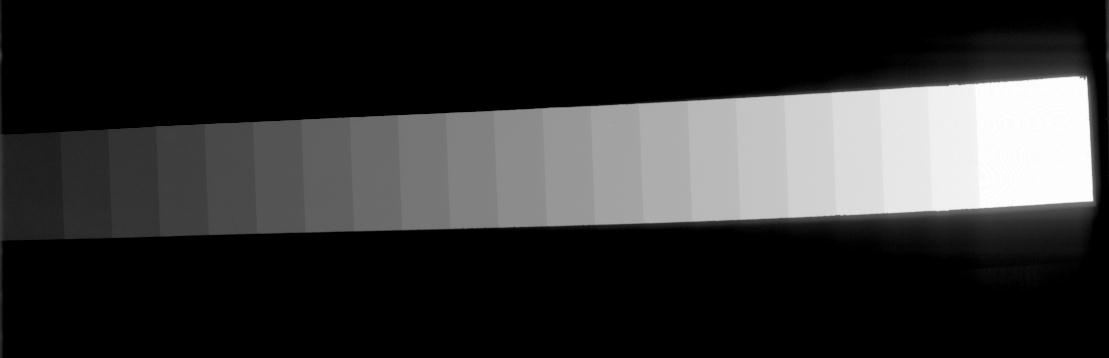## Mapping Grey Levels to Optical Density for the Lumisys Scanner

The scanner used at Wake Forest (formerly listed as Bowman Gray) was a Lumisys 200 laser densitometer. Cases with a filename beginning with the letters 'B' and 'C' came from WF. Cases from WF that have LUMISYS in the .ics file were scanned on the LUMISYS scanner at WF and can be calibrated to optical density with the equations in this file.

The Lumisys 200 laser densitometer at BGSM has the capacity to represent optical densities in the range from 0 to 3.6. Over this range, the digitizer uses 3600 different levels. It is obvious, that for this 12-bit machine, 3600 levels use only a portion of the range from 0 to 4095. Therefore, the machine is configured such that an OD of 0.0 corresponds to pixel value 4095. The 3600 grey levels start at this point (4095) and descend, matching each pixel value to an increment of 0.001 in OD.

Following this pattern to 3.6 OD gives a pixel value of 495 (ie. 4095 - 3600). Thus, the smallest values in digitized images from this scanner will be 495, not 0. The OD can be easily found from the pixel value by simply subtracting 495 from the measured pixel value, dividing the result by 1000, and subtracting this from 3.6. Here's the equation:

 Eq. 1 OD = 3.6 - (PixelValue - 495) / 1000

For example, a pixel value of 2478 corresponds to an OD of 1.617 (2478 - 495 = 1983; 1983/1000 = 1.983; 3.6 - 1.983 = 1.617).

If we measure the mapping of grey levels to optical density values experimentally, we get slightly different results. A step-wedge phantom film was scanned on the Lumisys scanner at a 210 micron per pixel spatial resolution. Here's a look at a cropped, rotated version of the phantom image.On the film, the optical density of each of the 21 wedges was measured as well as that of the film background. On the 210 micron image, the grey level mean and standard deviation of an 80 by 30 pixel region (240 pixels) within each wedge and the film background was computed. Here's the results of these measurements:

```   Measured  	  Grey Level Mean
Optical Density	(Standard Deviation)
---------------	--------------------
0.04	    4069 (14.2)
0.21	    3897 ( 8.7)
0.34	    3759 ( 6.2)
0.50	    3601 ( 5.2)
0.69	    3443 ( 5.5)
0.82	    3269 ( 5.7)
0.96	    3113 ( 5.2)
1.13	    2948 ( 4.0)
1.28	    2794 ( 6.2)
1.44	    2635 ( 5.2)
1.59	    2478 ( 5.2)
1.74	    2324 ( 5.4)
1.89	    2167 ( 5.5)
2.07	    2004 ( 5.8)
2.21	    1855 ( 7.6)
2.37	    1690 ( 7.9)
2.52	    1542 ( 9.5)
2.68	    1386 (12.3)
2.84	    1235 (15.4)
2.99	    1099 (21.7)
3.13	     957 (23.7)
3.60	     495 ( 0.0)
```

Here's a plot of mean grey level versus the measured optical density of the step wedge phantom. The error bars indicate the standard deviation of the grey levels within each wedge.Using simple regression to fit a line to the data, we get a slope of -1009.01, and a y-intercept of 4096.99. The equation for the line is:

y = -1009.01x + 4096.99

So, to convert a grey level (GL) value to optical density (OD), simply use the following equation:
 Eq. 2 OD = ( GL - 4096.99 ) / -1009.01

Example: Using Equation 2, a grey level of 2478 maps to an optical density of 1.6045. Looking at the table of measured OD values, we see the a grey level of 2478 mapped to a measured optical density of 1.59. Using the information provided with the Lumisys scanner (Eq. 1), a grey level of 2478 maps to an optical density of 1.617. The small discrepancies can be attributed to some combination of the noise introduced by the digitization process, and the noise in the experimental measurement of the OD function.